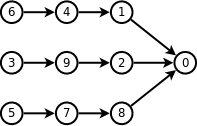# HDU - 4857 逃生 拓扑排序（反向建图）

HDU - 4857 逃生

Input

Output

Sample Input

1
5 10
3 5
1 4
2 5
1 2
3 4
1 4
2 3
1 5
3 5
1 2
Sample Output
1 2 3 4 5#include<iostream>
#include<algorithm>
#include<cstdlib>
#include<sstream>
#include<cstring>
#include<bitset>
#include<cstdio>
#include<string>
#include<deque>
#include<stack>
#include<cmath>
#include<queue>
#include<set>
#include<map>
#define mod 1000000007
using namespace std;
typedef long long ll;
const int maxn = 1e5+10;
struct node
{
int v;
int next;
}e[maxn];
int in[maxn];  //入度
int head[maxn];
int ans[maxn];
int n,m,cnt,tot;
void init()
{
cnt = tot = 0;
memset(in,0,sizeof(in));
memset(head,-1,sizeof(head));
}
void add(int u,int v)
{
e[cnt].v = v;
e[cnt].next = head[u];
head[u] = cnt++;
}
void tuopu()
{
priority_queue<int ,vector<int> ,greater<int> > q;   //大根堆
for(int i=1;i<=n;i++)
{
if(in[i]==0)
q.push(i);
}

while(!q.empty())
{
int u = q.top();
q.pop();
ans[tot++] = u;
for(int i=head[u] ; i!=-1 ; i=e[i].next)
{
int v = e[i].v;
in[v]--;
if(in[v]==0)
q.push(v);
}
}
}
int main()
{
int t;
int u,v;
scanf("%d",&t);
while(t--)
{
init();
scanf("%d%d",&n,&m);
for(int i=0;i<m;i++)
{
scanf("%d%d",&u,&v);
add(v,u);   //反向建图
in[u]++;
}
tuopu();
for(int i=tot-1;i>0;i--)
printf("%d ",ans[i]);
printf("%d\n",ans);

}

return 0;
}